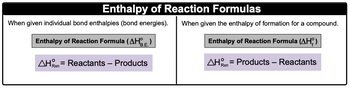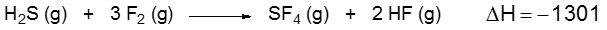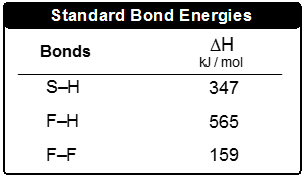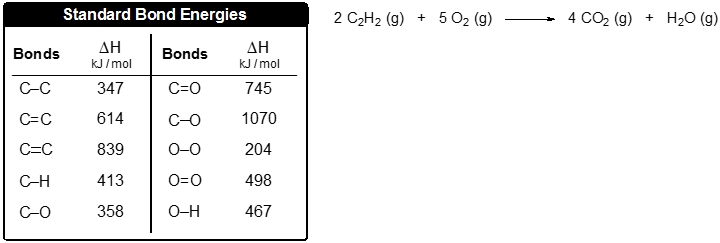## General Chemistry

Learn the toughest concepts covered in Chemistry with step-by-step video tutorials and practice problems by world-class tutors

11. Bonding & Molecular Structure

# Bond Energy

Bond Energy is the amount of energy stored within a chemical bond.

Bond Energy
1
concept

## Bond Energy1m
Play a video:
now here are going to say that bond energy, also called bond in therapy and it's represented by Delta H B E is the amount of energy stored in a bond between atoms in a molecule. Now realize that bond energy values can be calculated can be used to calculate the entropy of reaction, which is Delta h of reaction. Also, what's called the heat of reaction times as well. So just remember, it's the entropy, or heat of reaction. Now we have India thermic processes versus exile, thermic processes and in India, thermic process. Energy is absorbed toe break a bond. And since we're taking in energy where gaining energy, it has a positive sign in an extra thermic process. Energy is released, lost, and if you're losing energy, you have a negative sign. Now, if we take a look here, we're accustomed to seeing equations like this. These two equations you need to remember we're gonna say when given individual bond mantelpiece or bond energies, then the entropy of reaction formula becomes this Entropy of reaction equals reactant minus products. So again, if they're giving us individual bond anti appeaser bond energies, we use this version of the formula. Now, if you've looked at my videos in terms of thermal chemistry, you would see that that formula seems kind of familiar. Well, when we're given the entropy of formation for a whole compound, then its products minus react, it's so if you've seen my videos and thermal chemistry, you will remember this. If you haven't yet, don't worry about it just yet. Realize that when we're dealing with bond envelops bond energies, it's reactant minus products.

When individual bond energies are given, we will use the first formula.2
example

## Bond Energy Example 12m
Play a video:
here, it says the formation of ammonia is accomplished by the reaction between hydrogen and nitrogen gas. So here, it says, calculate the entropy of reaction. If the bond entropy Sorbonne Dental piece off the end. Triple bond, N H single bond, H and N Single Bond, H R. 9 45 for 32 3 91 killer jewels promote, respectively. So the steps we're gonna take here is we're gonna check to see if the chemical reaction is balanced. If and if not, then do the necessary steps to balance it. So here they already drawn for us. It's already balanced for us. We have one mole of this. If Lewis Structures is not given that you will have to draw them as well. Luckily, I gave it to us drawn. Now step one for the reactant and products multiply the coefficients of each bond type with its bond entropy value. All right, so if we take a look here we have one n triple bond and bond, and its energy is 9 45 kg per mole. And here we have three H single bond H bonds, and each one we're told is 4. 32 And then finally, we have Hominy and H funds. This one's tricky. We have one 23 n h bonds within NH three, but then it's times to so three times two equals six total N h bonds. So it's gonna be six and H bonds here. Each one is 3 91. So then what we need to realize here is that this will cancel out with the moles that we have and our aunts will be in killer jewels. So what we're gonna do here is 91 times 9 45 plus three times for 32 gives us 2 to +41 killer jewels here we're doing problem React. It's minus products to find the Delta h of reaction. Then we have six times 3 91 which is 2346 killer jewels. When we subtract thes two, it gives me negative 105 killer jewels as the entropy of reaction when given those different bond mantelpiece. So here all it is is first knowing that you have a balanced equation, knowing that you've drawn your Lewis dot structures and carefully examining the different types of bonds that exist. If you could do that, you'll be able to find the entropy of reaction for any of these given questions
3
Problem

Consider the following equation:Determine the bond enthalpy value for the F–S bond.4
Problem

Use the bond energies to estimate the enthalpy of reaction for the combustion of 5 moles of acetylene: